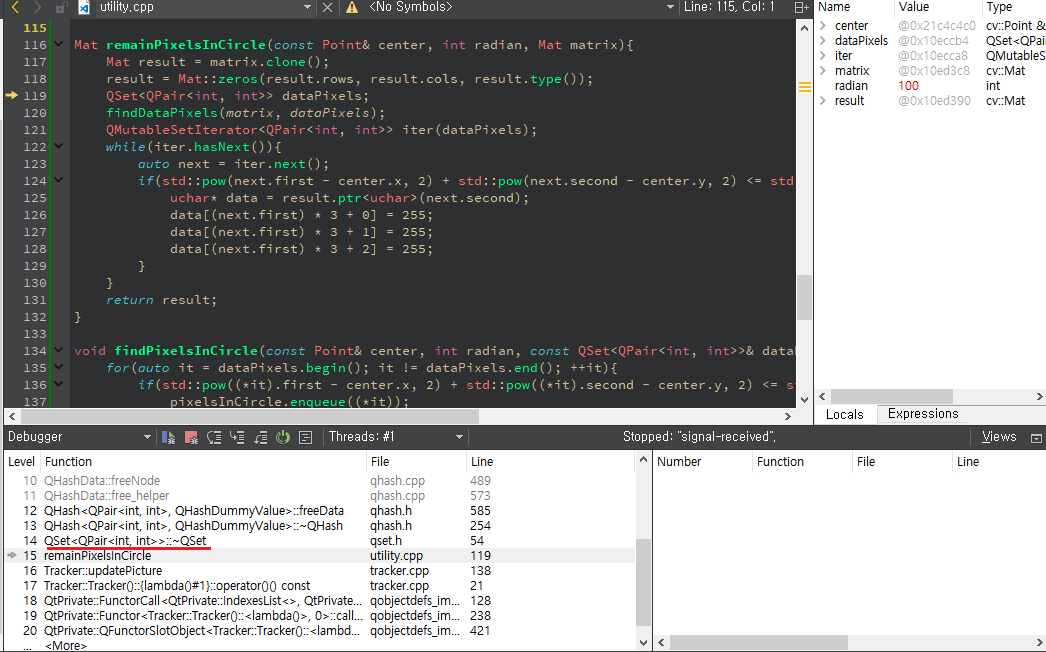# A signal was received from inner QHash

•``````Mat remainPixelsInCircle(const Point& center, int radian, Mat matrix){
Mat result = matrix.clone();
result = Mat::zeros(result.rows, result.cols, result.type());
QSet<QPair<int, int>> dataPixels;
findDataPixels(matrix, dataPixels);
QMutableSetIterator<QPair<int, int>> iter(dataPixels);
while(iter.hasNext()){
auto next = iter.next();
if(std::pow(next.first - center.x, 2) + std::pow(next.second - center.y, 2) <= std::pow(radian, 2)){
uchar* data = result.ptr<uchar>(next.second);
data[(next.first) * 3 + 0] = 255;
data[(next.first) * 3 + 1] = 255;
data[(next.first) * 3 + 2] = 255;
}
}
return result;
}
``````

It seems that the signal was sent from a destructor of QSet. But I have no idea what happens inside.

• Why do you use QMutableSetIterator? I don't see where you modify the set...

• @Asperamanca I left it even after I removed the code part which modifies the set. I solved it after I changed it to normal iterator. Thanks!